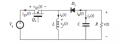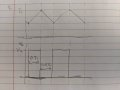# Buck Boost Converter MOSFET Voltage #2

#### savdowning

Joined Feb 18, 2022
2
Have you figured this out yet?

Think about what the voltage across the inductor has to be when the switch is on and with the switch is off when the converter operates in CCM.
Hello, I am having trouble with this part. I am completely following until finding the DC component of the fet voltage. Can you explain what you said here a little more and how it pertains to the average voltage across the mosfet?

#### MrAl

Joined Jun 17, 2014
8,992
Hello, I am having trouble with this part. I am completely following until finding the DC component of the fet voltage. Can you explain what you said here a little more and how it pertains to the average voltage across the mosfet?
Well at the surface this is not too hard to understand but you do have to be able to calculate the voltage on both sides of the switch.
On the left side we have the DC input so that's not too hard to figure out, it's the battery voltage.
On the right side however we have to be able to calculate the wave shape using circuit analysis techniques.
Once we have the voltage on both sides of the switch vLeft and vRight, we can subtract to get the wave across the switch in time:
vSwitch=vLeft-vRight
but you should be able to do vRight=vLeft also. They will both be functions of time but of course the battery voltage is just a constant assuming zero internal resistance.
Next you apply the integral for the average voltage and that givers you the average DC voltage across the switch.

There may be a shortcut here but we are probably better off doing it this way as this applies to any circuit no matter how it is hooked up.

•savdowning

#### MrAl

Joined Jun 17, 2014
8,992
This new thread is kind of nutty. There's no circuit to go with the additional help.
It was originally just a reply to a thread i had replied to before so not sure why it would be moved to a new thread with no way to let anyone know what was being discussed.
Should i maybe post a schematic or something?

#### ericgibbs

Joined Jan 29, 2010
15,526
hi Al,
EWorking on a homework on buck-boost, given the following :
• Vin = 12V
• Vout = -5V
• R load = 2.5 ohm
• Fsw = 20kHz
• Assume ideal, at CCM
I have calculated more values based on some of the questions it asked:
1. Duty cycle = 0.2941
2. Inductor current = 2.833A
3. Inductor value (assuming delta IL = 15% of IL) = 20.76uH
4. Capacitor value (assuming delta Vc = 25mV) = 58.82uF
I am having trouble answering the last 2 questions of this HW:My understanding is that the Vq is the switching voltage. Which means that the waveform should look something like this:Assuming that, for question 12, the peak voltage should be equal to Vin = 12V. Furthermore, for #13, my guess is the DC component of this waveform should be Vin * D = 2.99V. Of course I got both questions wrong and thus I'm here looking for advice. Not sure how else to go about this logic at the moment. Any help is appreciated, thanks!

#### MrAl

Joined Jun 17, 2014
8,992
hi Al,
E

View attachment 261125

Working on a homework on buck-boost, given the following :
• Vin = 12V
• Vout = -5V
• R load = 2.5 ohm
• Fsw = 20kHz
• Assume ideal, at CCM
I have calculated more values based on some of the questions it asked:
1. Duty cycle = 0.2941
2. Inductor current = 2.833A
3. Inductor value (assuming delta IL = 15% of IL) = 20.76uH
4. Capacitor value (assuming delta Vc = 25mV) = 58.82uF
I am having trouble answering the last 2 questions of this HW:My understanding is that the Vq is the switching voltage. Which means that the waveform should look something like this:Assuming that, for question 12, the peak voltage should be equal to Vin = 12V. Furthermore, for #13, my guess is the DC component of this waveform should be Vin * D = 2.99V. Of course I got both questions wrong and thus I'm here looking for advice. Not sure how else to go about this logic at the moment. Any help is appreciated, thanks!
Oh that's great Eric. Not sure what a co-mod is though